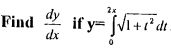【站僕】摩檸Morning>試卷(2016/09/05)

# 97 年 - 97 淡江大學 轉學考 高等微積分#55794

【非選題】
1.1. Show that t(x) =is not uniformly continuous on (0,1). (10 points).

【非選題】
2.2.【非選題】
3.3. Iff: R is differentiable and i = f(x-y), show that=0.(10 points)

【非選題】
4.4. Let K be a compact subset of R and let f be a real —vaiued function on K. Prove that if f is continuous on K, then f (K) is compact. (10 points)

【非選題】
5.
5. Letf(x, y)= (xy ,x2 + y2).

【題組】(1). Show that g=f-1 exists and is differentiable in some nonempty open set containing (2,5). (10 points)

【非選題】
6.【題組】(2). Find Dg(2，5)(the total derivative ofg at(2,5)). (10 points)

【非選題】
7.6. Let f be continuous on [a, b]. Show thatA in [a, b] if and only iff(x) = 0 for all x in[a，b]. (15 points)

【非選題】
8.7. Let t : [0, l]→[0，1] be continuous. Prove that there is c[0，11 sucli that f(c)=c. (10 points)

【非選題】
9.8.(5 points)

【非選題】
10.9. Let {fn} be a sequence of real-vaiued functions on [0，l] and  fn converges uniformly to a function f. Prove that if each fn is continuous on [0,1], then f is continuous on [0,1]. (10 points)

### 懸賞詳解

#### 國二自然上第一次

32.將稀鹽酸滴在（甲）砂岩（乙）板岩（丙）安山岩（丁）石灰岩（戊）大理岩 岩石 標本上，請問有幾種會產生氣泡? 【題組】33.承32題，將上�...